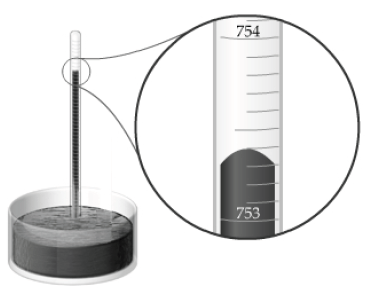# Problem: The barometer shown here measures atmospheric pressure in millimeters of mercury (mmHg). According to this barometer, what is the atmospheric pressure P in inches of mercury (inHg)?Express your answer in inches of mercury using five significant figures.Air pressure is measured by a barometer. One type of barometer utilizes an evacuated, calibrated tube inverted in a pool of mercury. The pool of mercury is open to the atmosphere. As air pressure increases, the mercury will rise in the column until the weight of the mercury in the column equals the force exerted by the air on the surface of the pool. The difference in height between the top of the mercury in the column and level of mercury in the pool is directly proportional to air pressure and quantitatively measures air pressure. Such columns are often calibrated in units of millimeters and thus the pressure read from such a column is reported as mm of Hg, abbreviated mmHg, since the liquid in the column is mercury.Units of pressureThe column of a mercury barometer is calibrated in linear units (such as inches or millimeters) and thus the air pressure observed on such a barometer is reported in terms of these linear units. This measurement of pressure can be converted to other common pressure units. Some conversion factors are shown here.1 mmHg = 1 torr1 atm = 760 mmHg1 atm = 1.01325 bar1 Pa = 10−5 bar1 in = 2.54 cm1 cm = 10 mm

###### FREE Expert SolutionView Complete Written Solution
###### Problem Details

The barometer shown here measures atmospheric pressure in millimeters of mercury (mmHg). According to this barometer, what is the atmospheric pressure P in inches of mercury (inHg)?Air pressure is measured by a barometer. One type of barometer utilizes an evacuated, calibrated tube inverted in a pool of mercury. The pool of mercury is open to the atmosphere. As air pressure increases, the mercury will rise in the column until the weight of the mercury in the column equals the force exerted by the air on the surface of the pool. The difference in height between the top of the mercury in the column and level of mercury in the pool is directly proportional to air pressure and quantitatively measures air pressure. Such columns are often calibrated in units of millimeters and thus the pressure read from such a column is reported as mm of Hg, abbreviated mmHg, since the liquid in the column is mercury.

Units of pressure

The column of a mercury barometer is calibrated in linear units (such as inches or millimeters) and thus the air pressure observed on such a barometer is reported in terms of these linear units. This measurement of pressure can be converted to other common pressure units. Some conversion factors are shown here.

1 mmHg = 1 torr
1 atm = 760 mmHg
1 atm = 1.01325 bar
1 Pa = 10−5 bar
1 in = 2.54 cm
1 cm = 10 mm